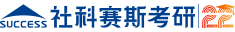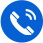400-8030-969

24h统一客服热线

# 别再发愁MPAcc联考中的数列类难题

### 2023管理类联考备考大礼包限时领取中...

基本数列是等差数列和等比数列

一、等差数列

一个等差数列由两个因素确定：首项a1和公差d。

得知以下任何一项，就可以确定一个等差数列(即求出数列的通项公式)：

1、首项a1和公差d

2、数列前n项和s(n)，因为s(1)=a1，s(n)-s(n-1)=a(n)

3、任意两项a(n)和a(m)，n，m为已知数

等差数列的性质：

1、前N项和为N的二次函数(d不为0时)

2、a(m)-a(n)=(m-n)*d

3、正整数m、n、p为等差数列时，a(m)、a(n)、a(p)也是等差数列

例题1：已知a(5)=8，a(9)=16，求a(25)

解： a(9)-a(5)=4*d=16-8=8

a(25)-a(5)=20*d=5*4*d=40

a(25)=48

例题2：已知a(6)=13，a(9)=19，求a(12)

解：a(6)、a(9)、a(12)成等差数列

a(12)-a(9)=a(9)-a(6)

a(12)=2*a(9)-a(6)=25

二、等比数列

一个等比数列由两个因素确定：首项a1和公差d。

得知以下任何一项，就可以确定一个等比数列(即求出数列的通项公式)：

1、首项a1和公比r

2、数列前n项和s(n)，因为s(1)=a1，s(n)-s(n-1)=a(n)

3、任意两项a(n)和a(m)，n，m为已知数

等比数列的性质：

1、a(m)/a(n)=r^(m-n)

2、正整数m、n、p为等差数列时，a(m)、a(n)、a(p)是等比数列

3、等比数列的连续m项和也是等比数列

即b(n)=a(n)+a(n+1)+。。。+a(n+m-1)构成的数列是等比数列。

三、数列的前N项和与逐项差

1、如果数列的通项公式是关于N的多项式，最高次数为P，则数列的前N项和是关于N的多项式，最高次数为P+1。(这与积分很相似)

2、逐项差就是数列相邻两项的差组成的数列。

如果数列的通项公式是关于N的多项式，最高次数为P，则数列的逐项差的通项公式是关于N的多项式，最高次数为P-1。

(这与微分很相似)

例子：

1，16，81，256，625，1296 (a(n)=n^4)

15，65，175，369，671

50，110，194，302

60，84，108

24，24

从上例看出，四次数列经过四次逐项差后变成常数数列。

等比数列的逐项差还是等比数列

四、已知数列通项公式A(N)，求数列的前N项和S(N)。

这个问题等价于求S(N)的通项公式，而S(N)=S(N-1)+A(N)，这就成为递推数列的问题。

解法是寻找一个数列B(N)，

使S(N)+B(N)=S(N-1)+B(N-1)

从而S(N)=A(1)+B(1)-B(N)

猜想B(N)的方法：把A(N)当作函数求积分，对得出的函数形式设待定系数，利用B(N)-B(N-1)=-A(N)求出待定系数。

题1：求S(N)=2+2*2^2+3*2^3+。。。+N*2^N

解：S(N)

=S(N-1)+N*2^N

N*2^N积分得(N*LN2-1)*2^N/(LN2)^2

因此设B(N)=(PN+Q)*2^N

则 (PN+Q)*2^N-[P(N-1)+Q)*2^(N-1)=-N*2^N

(P*N+P+Q)/2*2^N=-N*2^N

因为上式是恒等式，所以P=-2，Q=2

B(N)=(-2N+2)*2^N

A(1)=2，B(1)=0

因此：S(N)=A(1)+B(1)-B(N)=(2N-2)*2^N+2

题2：A(N)=N*(N+1)*(N+2)，求S(N)

解法1：S(N)为N的四次多项式，

设：S(N)=A*N^4+B*N^3+C*N^2+D*N+E

利用S(N)-S(N-1)=N*(N+1)*(N+2)

解出A、B、C、D、E

解法2：

S(N)/3!=C(3，3)+C(4，3)+。。。C(N+2，3)=C(N+3，4)

S(N)=N*(N+1)*(N+2)*(N+3)/4X